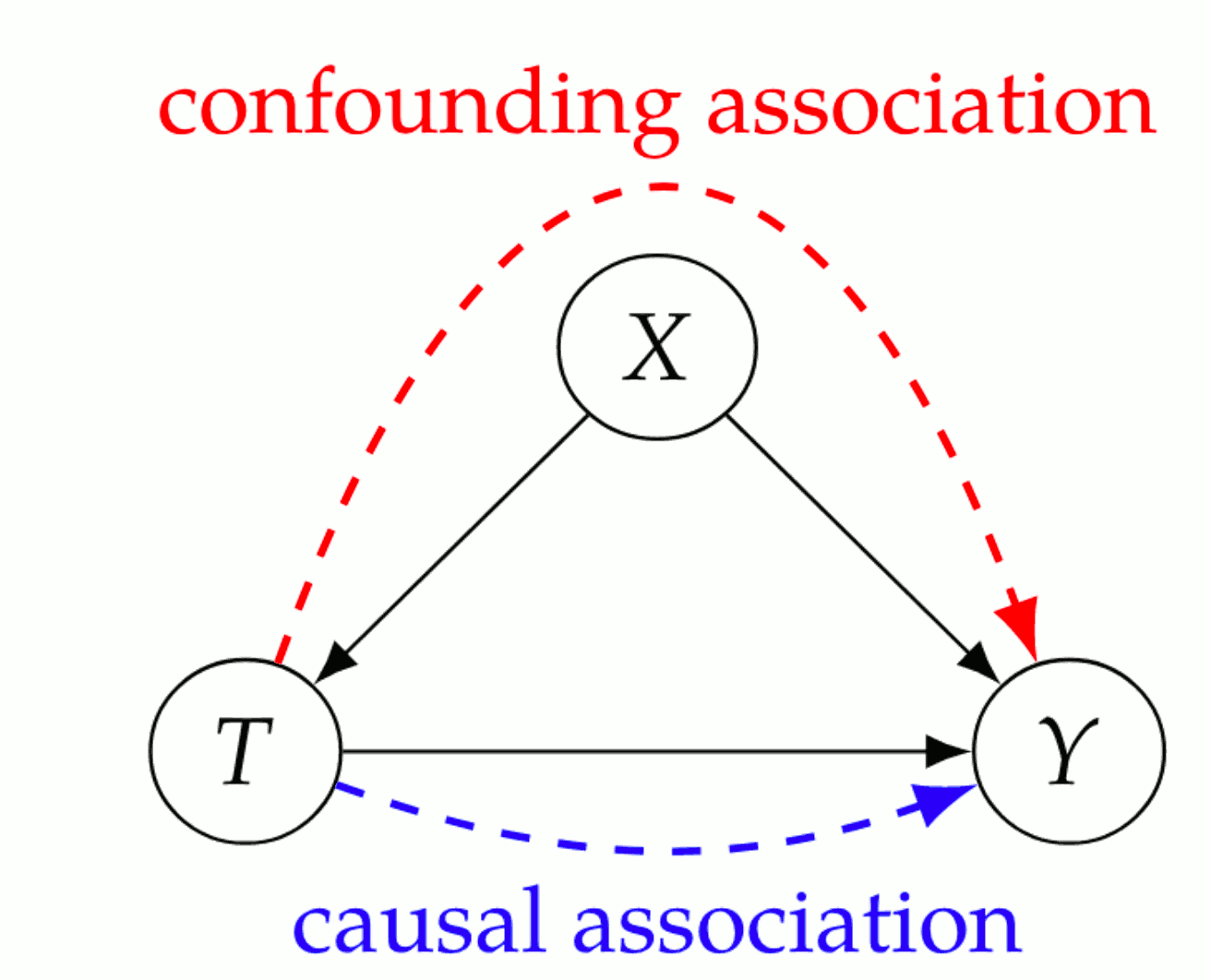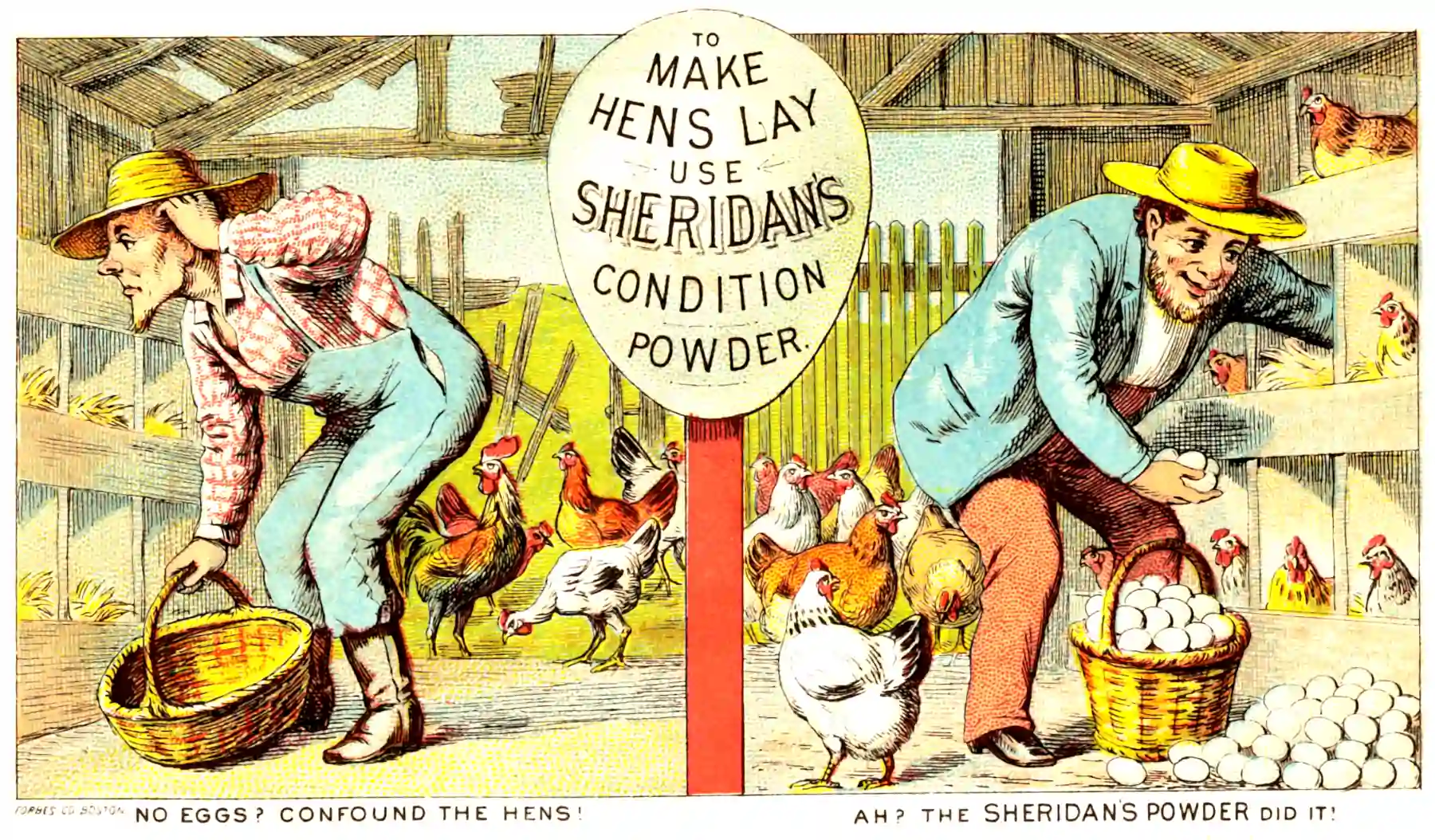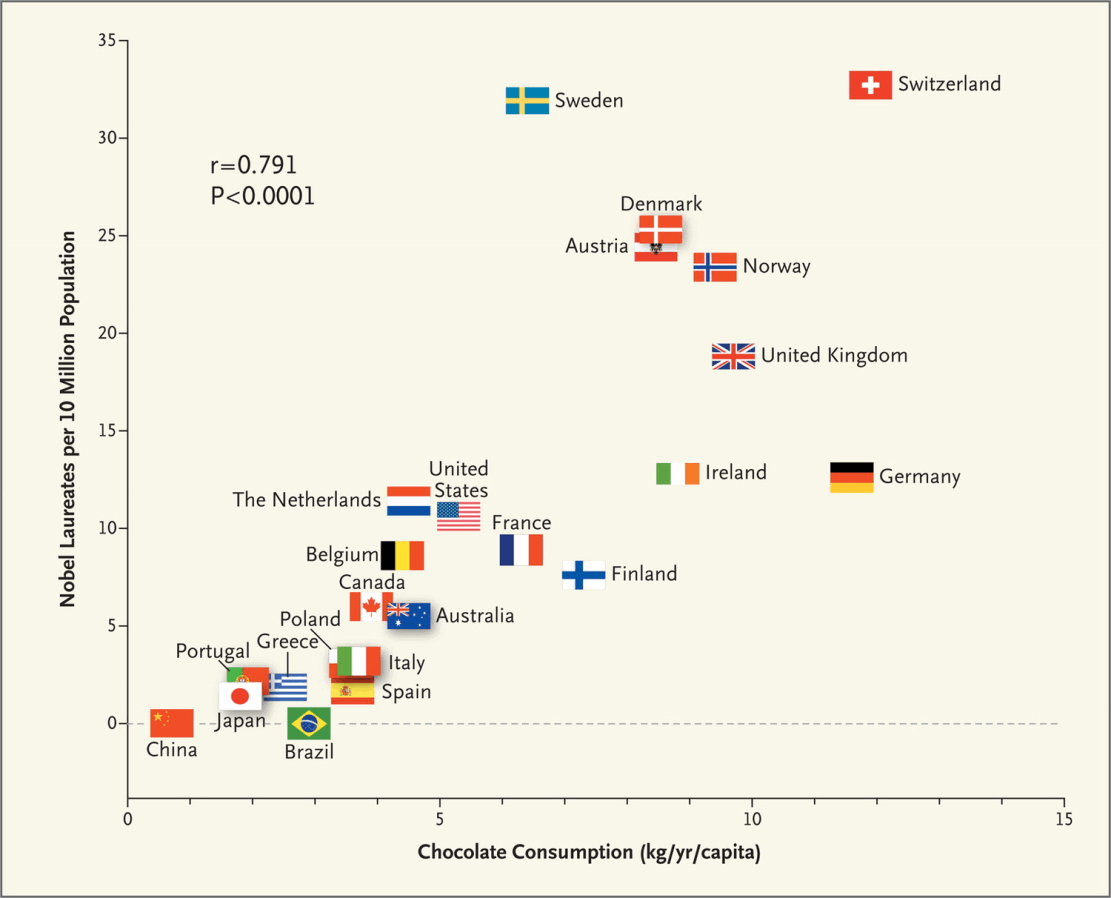# Causal graphical model reading group 2022

## Causal inference

$\renewcommand{\var}{\operatorname{Var}} \renewcommand{\corr}{\operatorname{Corr}} \renewcommand{\dd}{\mathrm{d}} \renewcommand{\vv}{\boldsymbol{#1}} \renewcommand{\rv}{\mathsf{#1}} \renewcommand{\vrv}{\vv{\rv{#1}}} \renewcommand{\disteq}{\stackrel{d}{=}} \renewcommand{\gvn}{\mid} \renewcommand{\Ex}{\mathbb{E}} \renewcommand{P}{\mathbb{P}} \renewcommand{\indep}{\mathop{\perp\!\!\!\perp}}$

My chunk (Chapter 3) of the internal reading group covering the Brady Neal course.

## Recap: potential outcomes

Last time we discussed the potential outcome framework, which answers the question: How do we calculate a treatment effect $$Y(t)$$ for some treatment $$t$$? i.e. how do we calculate $\Ex[Y(1)-Y(0)]?$

We used the following assumptions: \begin{aligned} (Y(1), Y(0))\indep T | X &\quad \text{Unconfoundedness}\\ Y = Y(t)&\quad \text{Consistency}\\ (Y(1), Y(0))\indep T&\quad \text{Overlap}\\ (Y(1), Y(0))\indep T&\quad \text{No interference}\\ \end{aligned}

Under those assumptions, we have the causal adjustment formula $\Ex[Y(1) − Y(0)] = \Ex_{X}\left[ \Ex[Y | T{=}t, X] − \Ex[Y | T{=}s, X]\right].$

Aside: what is going on in positivity?

And now…

## Graphical models for causation wrangling

We have a finite collections of random variables $$\mathbf{V}=\{X_1, X_2,\dots\}$$.

For simplicity of exposition, each of the RVs will be discrete so that we may work with pmfs, and write $$P(X_i|X_j)$$ for the pmf. I sometimes write $$P(x_i|x_j)$$ to mean $$P(X_i=x_i|X_j=x_j)$$.

More notation. We write $X \indep Y|Z$ to mean “$$X$$ is independent of $$Y$$ given $$Z$$”.

We can solve these questions via a graph formalism. That’s where the DAGs come in.

### Directed Acyclic Graphs (DAGs)

A DAG is graph with directed edges, and no cycles. (you cannot return to the same starting node travelling only forward along the arrows.)

DAGs are defined by a set of vertexes and (directed) edges.

We show the directions of edges by writing them as arrows.

For nodes $$X,Y\in \mathbf{V}$$ we write $$X \rightarrow Y$$ to mean there is a directed edged joining them.

## Bayesian networks

### Local Markov assumption

Given its parents in the DAG, a node is independent of all its non-descendants.

With four variable example, the chain rule of probability tells us that we can factorize any $$P$$ thus $P\left(x_{1}, x_{2}, x_{3}, x_{4}\right)=P\left(x_{1}\right) P\left(x_{2} \mid x_{1}\right) P\left(x_{3} \mid x_{2}, x_{1}\right) P\left(x_{4} \mid x_{3}, x_{2}, x_{1}\right) \text {. }$Figure 1: Abstract DAG

If $$P$$ is Markov with respect to the above graph then we can simplify the last factor: $P\left(x_{1}, x_{2}, x_{3}, x_{4}\right)=P\left(x_{1}\right) P\left(x_{2} \mid x_{1}\right) P\left(x_{3} \mid x_{2}, x_{1}\right) P\left(x_{4} \mid x_{3}\right) .$

If we further remove edges, removing $$X_{1} \rightarrow X_{2}$$ and $$X_{2} \rightarrow X_{3}$$ as the below figure,Figure 2: Abstract DAG 2

we can further simplify the factorization of $$P$$ : $P\left(x_{1}, x_{2}, x_{3}, x_{4}\right)=P\left(x_{1}\right) P\left(x_{2}\right) P\left(x_{3} \mid x_{1}\right) P\left(x_{4} \mid x_{3}\right)$

### Bayesian Network Factorization

Given a probability distribution $$P$$ and a DAG $$G$$, we say $$P$$ factorizes according to $$G$$ if $P\left(x_{1}, \ldots, x_{n}\right)=\prod_{i} P\left(x_{i} \mid \operatorname{parents}(X_i)\right).$

### Minimality

1. Given its parents in the DAG, a node X is independent of all its non-descendants
2. Adjacent nodes in the DAG are dependent.

## Causal interpretationCausal association from Neal (2020)

### Causal Edges

In a directed graph, every parent is a direct cause of all its children.

$$Y$$ “directly causing“ $$X$$ means that a $$X=f(\operatorname{parent}(X),\omega)$$ is a (stochastic) function of some parent set which includes $$Y,$$ and some independent noise.

### Causal Bayesian Networks

Causal Edges + Local Markov

## Conditional independence in Bayesian networks

When we fix some nodes, which independences do we introduce?### ChainsB in a chain path

$P(a,b,c) = P(a)P(b|a)P(c|b)$

We assert that, conditional on B, A and C are independent: $A\indep C | B \\ \Leftrightarrow\\ P(a,c|b) = P(a|b)P(c|b)$

In slow motion, \begin{aligned} P(a,b,c) &= P(a)P(b|a)P(c|b)\\ P(a,c|b) &=\frac{P(a)P(b|a)P(c|b)}{P(b)}\\ &=P(c|b)\frac{P(a)P(b|a)}{P(b)}\\ &=P(c|b)\frac{P(a,b)}{P(b)}\\ &=P(c|b)P(a|b) \end{aligned}

### ForksB in a fork path

$P(a,b,c) = P(b)P(a|b)P(c|b)$

We assert that, conditional on B, A and C are independent: $A\indep C | B \\ \Leftrightarrow\\ P(a,c|b) = P(a|b)P(c|b)$ In slow motion, \begin{aligned} P(a,b,c) &= P(b)P(a|b)P(c|b)\\ P(a,c|b) &=\frac{P(b)P(a|b)P(c|b)}{P(b)}\\ &=P(a|b)P(c|b) \end{aligned}

### Immoralities

(Colliders when I grew up.)B in a collider path

$P(a,b,c) = P(b)P(c)P(a|b,c)$

We assert that, conditional on B, A and C are not in general independent: $A \cancel{\indep} C | B \\ \Leftrightarrow\\ P(a,c|b) = P(a|b)P(c|b)$

Proof that this never factorizes?

### Blocked paths

A path between nodes $$X$$ and $$Y$$ is blocked by a (potentially empty) conditioning set $$Z$$ if either of the following is true:

1. Along the path, there is a chain $$\cdots \rightarrow W \rightarrow \cdots$$ or a fork $$\cdots \leftarrow W \rightarrow \cdots$$, where $$W$$ is conditioned on $$(W \in Z)$$.
2. There is a collider $$W$$ on the path that is not conditioned on $$(W \notin Z)$$ and none of its descendants are conditioned on $$(\operatorname{descendants}(W) \nsubseteq Z)$$.

### d-separation

Two (sets of) nodes $$\vv{X}$$ and $$\vv{Y}$$ are $$d$$-separated by a set of nodes $$\vv{Z}$$ if all of the paths between (any node in) $$\vv{X}$$ and (any node in) $$\vv{Y}$$ are blocked by $$\vv{Z}$$.

### d-separation in Bayesian networks

We use the notation $$X \indep_{G} Y \mid Z$$ to denote that $$X$$ and $$Y$$ are d-separated in the graph $$G$$ when conditioning on $$Z$$. Similarly, we use the notation $$X \indep_{P} Y \mid Z$$ to denote that $$X$$ and $$Y$$ are independent in the distribution $$P$$ when conditioning on $$Z$$.

Given that $$P$$ is Markov with respect to $$G$$ if $$X$$ and $$Y$$ are d-separated in $$G$$ conditioned on $$Z$$, then $$X$$ and $$Y$$ are independent in $$P$$ conditioned on $$Z$$.

$X \indep_{G} Y |Z \Longrightarrow X \indep_{P} Y | Z.$

# d-separation implies Association is CausationChocolate and Nobel prizes, Messerli (2012)Correlation tastes as good as causation.

### No comments yet. Why not leave one?

GitHub-flavored Markdown & a sane subset of HTML is supported.